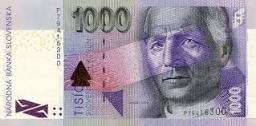# Saleswoman 2667

Martin bought goods in the store for 260 CZK. He paid with a thousand CZK crowns. The saleswoman returned 18 banknotes and coins worth CZK 10 and CZK 50. How many were there?

a =  4
b =  14

### Step-by-step explanation:

a+b = 18
10a + 50b = 1000-260

a+b = 18
10•a + 50•b = 1000-260

a+b = 18
10a+50b = 740

a = 4
b = 14

Our linear equations calculator calculates it.Did you find an error or inaccuracy? Feel free to write us. Thank you!

Tips for related online calculators
Do you have a system of equations and looking for calculator system of linear equations?